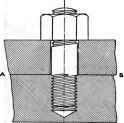### Notation

The following notation la used throughout the charter on Bolts, Studs, Nuts, and Screws: d = Diameter of bolt (Inches).

d\ = Diameter at root of thread (inches).

H = Height of nut (inches).

I = Initial axial tension (lbs.).

k = Allowable bearing pressure on surface of thread (lbs. per sq. in.).

L = Lead, or distance nut advances along axis in one revolution (inches).

/ = Length of wrench handle (inches) n = Number of threads in nut.

p = Pitch of thread, or distance between similar points on adjacent threads, measured parallel to axis (inches).

S = Fiber stress (lbs. per sq. in.).

W = Load on bolt (lbs.).Fig. 54.

### Analysis

A bolt is simply a cylindrical bar of metal upset at one end to form a head, and having a thread at the other end, Fig. 54. A stud is a bolt in which the head is replaced by a thread; or it is a cylindrical bar threaded at both ends, usually having a small plain portion in the middle, Fig. 55. The object of bolts and studs is to clamp machine parts together, and yet permit these same parts to be readily disconnected. The bolt passes through the pieces to be connected, and, when tightened, causes surface compression between the parts, while the reactions on the head and nut produce tension in the bolt. Studs and tap bolts pass through one of the connected parts and are screwed into the other, the stud remaining in position when the parts are disconnected.

As all materials are elastic within certain limits, the action of a bolt in clamping two machine parts together, more especially if there is an elastic packing between them, may be represented diagrammatically by Fig. 56, in which a spring has been introduced to take the compression due to screwing up the nut. Evidently the tension in the bolt is equal to the force necessary to compress the spring. Now, suppose that two weights, each equal to ½ W, are placed symmetrically on either side of the bolt, then the tension in the bolt will be increased by the added weights if the bolt is perfectly rigid. The bolt, however, stretches; hence some of the compression on the spring is relieved and the total tension in the bolt is less than W + I, by an amount depending on the relative elasticity of the bolt and spring.Fig. 55.Fig. 55a.

Suppose that the stud in Fig. 55 is one of the studs connecting the cover to the cylinder of a steam engine, and that the studs have a small initial tension; then the pressure of the steam loads each stud, and, if the studs stretch enough to relieve the initial pressure between the two surfaces, then their stress is due to the steam pressure only; or, from Fig. 56, when I = W ; the initial pressure due to the elasticity of the joint is entirely relieved by the assumed stretch of the studs. Except to prevent leakage, it is seldom necessary to consider the initial tension, for the stretch of the bolt may be counted on to relieve this force, and the working tension on the bolt is simply the load applied.

For shocks or blows, as in the case of the bolts found on the marine type of connecting-rod end, the stretch of the bolts acts like a spring to reduce the re-suiting tensions. So important is this feature that the body of the bolt is frequently turned down to the diameter of the bottom of the thread, thus uniformly distributing the stretch through the full length of the bolt, instead of localizing it at the threaded parts.

In tightening up a bolt, the friction at the surface of the thread produces a twisting moment, which increases the stress in the bolts, just as in the ease of shafting under combined tension and torsion; but the increase is small in amount, and may readily be taken care of by permitting low values only for the fiber stress.

In a flange coupling, bolts are acted upon by forces perpendicular to the axis, and hence are under pure shearing stress. If the torque on the shaft becomes too great, failure will occur by the bolts shearing off at the joint of the coupling.

A bolt under tension communicates its load to the nut through the locking of the threads together. If the nut is thin, and the number of threads to take the load few, the threads may break of shear off at the root. With a V thread there is produced a component force, perpendicular to the axis of the bolt, which tends to split the nut.Fig. 56.

In screws for continuous transmission of motion and power, the thread may be compared to a rough inclined plane, on which a small block, the nut, is being pushed upward by a force parallel to the base of the plane. The angle at the bottom of the pl'sane is the angle of the helix, or an angle whose tangent is the lead divided by the circumference of the screw. The horizontal force corresponds to the tangential force on the screw. The friction at the surface of the thread produces a twisting moment about the axis of the screw, which, combined with the axial load, subjects the screw to combined tension and torsion. Screws with square threads are generally used for this service, the sides of the thread exerting no bursting pressure on the nut. The proportions of screw thread for transmission of power depend more on the bearing pressure than on strength. If the bearing surface be too small and lubrication poor, the screw will cut and wear rapidly.